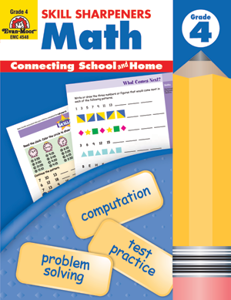# Skill Sharpeners Math, Grade 4

EMC4548
Regular price \$9.99 \$0.00

Skill Sharpeners Math, Grade 4 provides practice for the following mathematics skills:

• computation using addition, subtraction, mulitplication, and division
• decimals
• fractions
• rounded numbers & estimation
• place value
• factors, multiples, prime numbers
• area and perimeter
• symmetry
• graphing, grids, charts, and tables
• money & time
• linear measurement, weight and temperature
• time
• logic puzzles